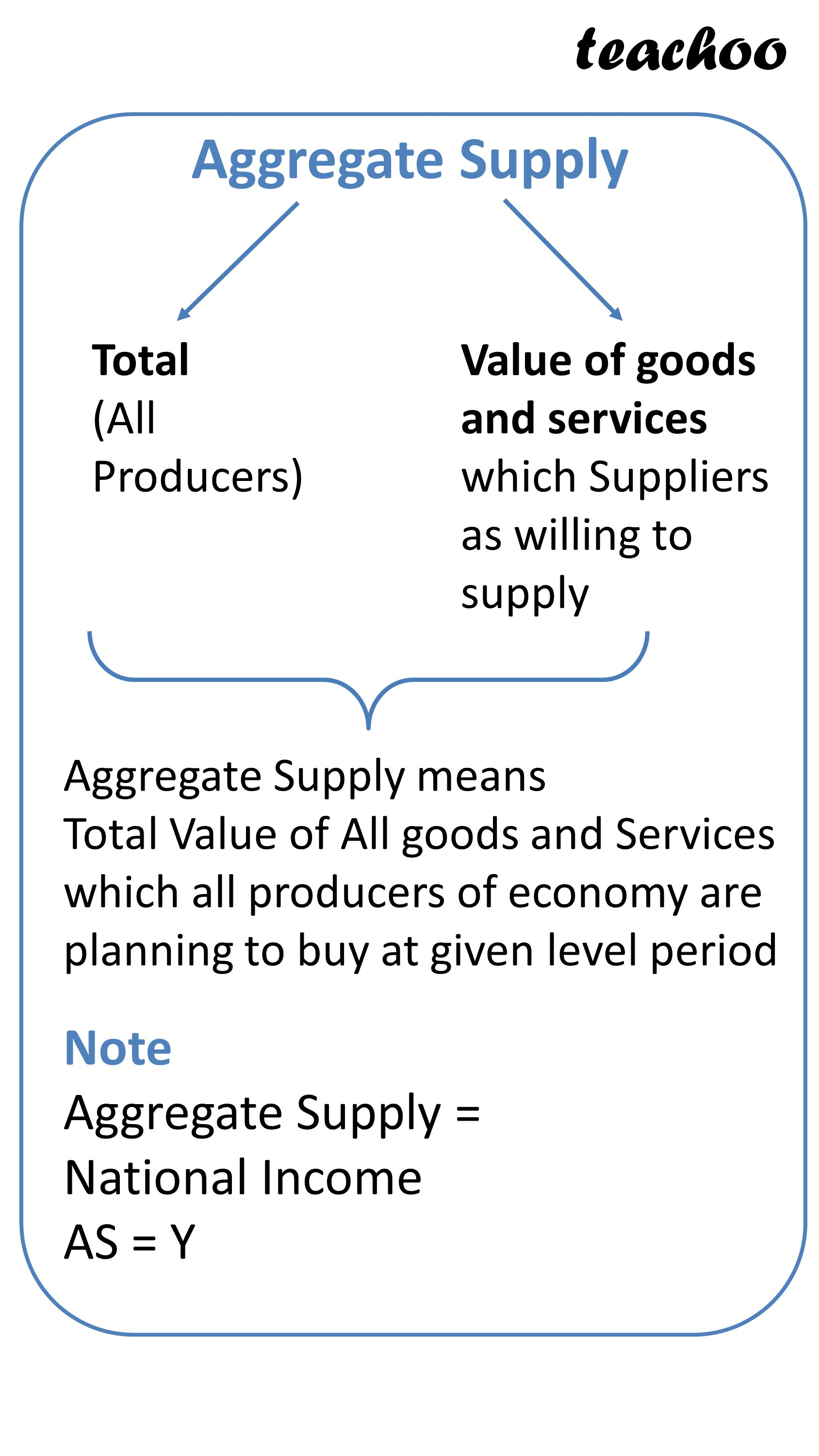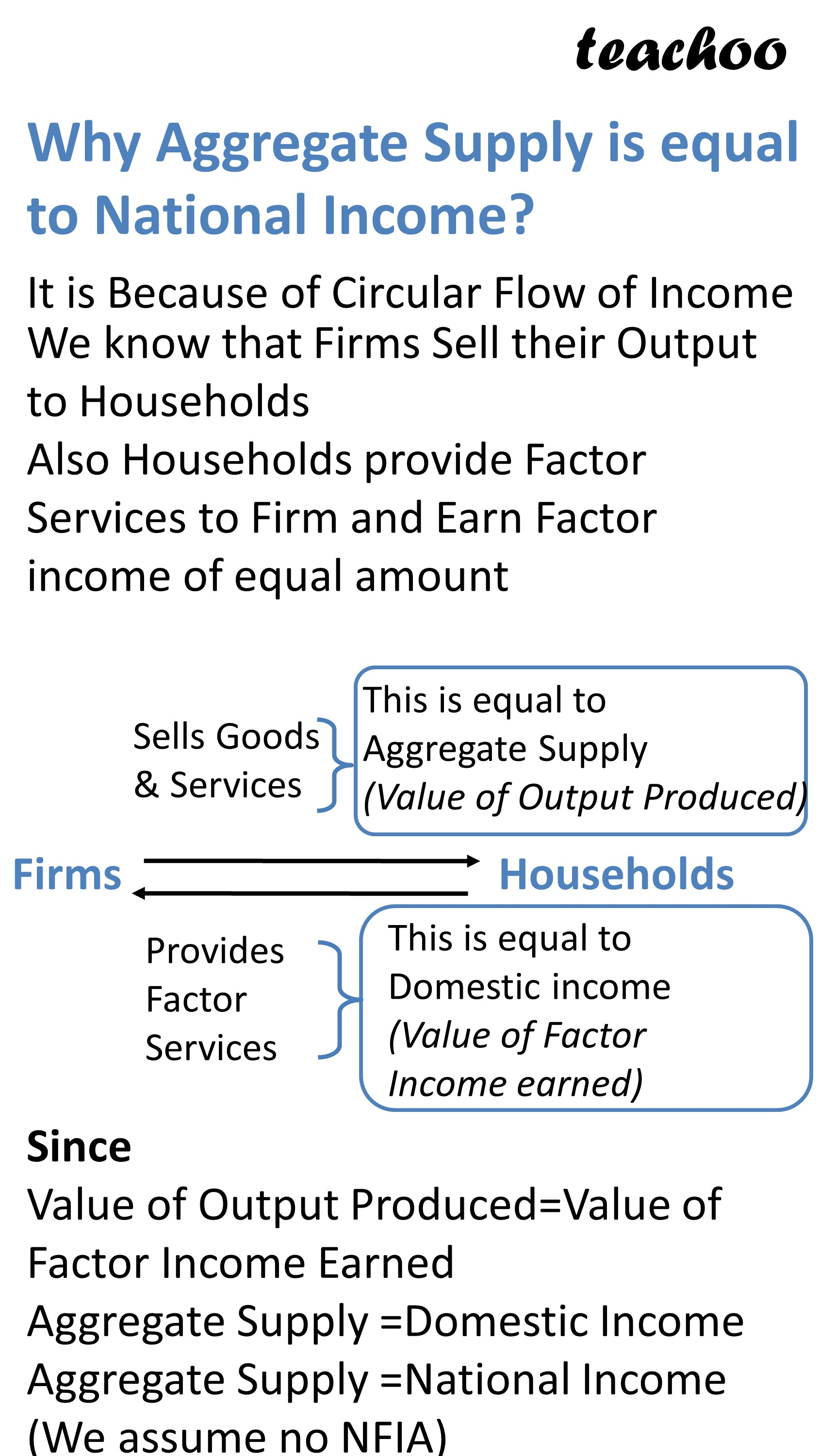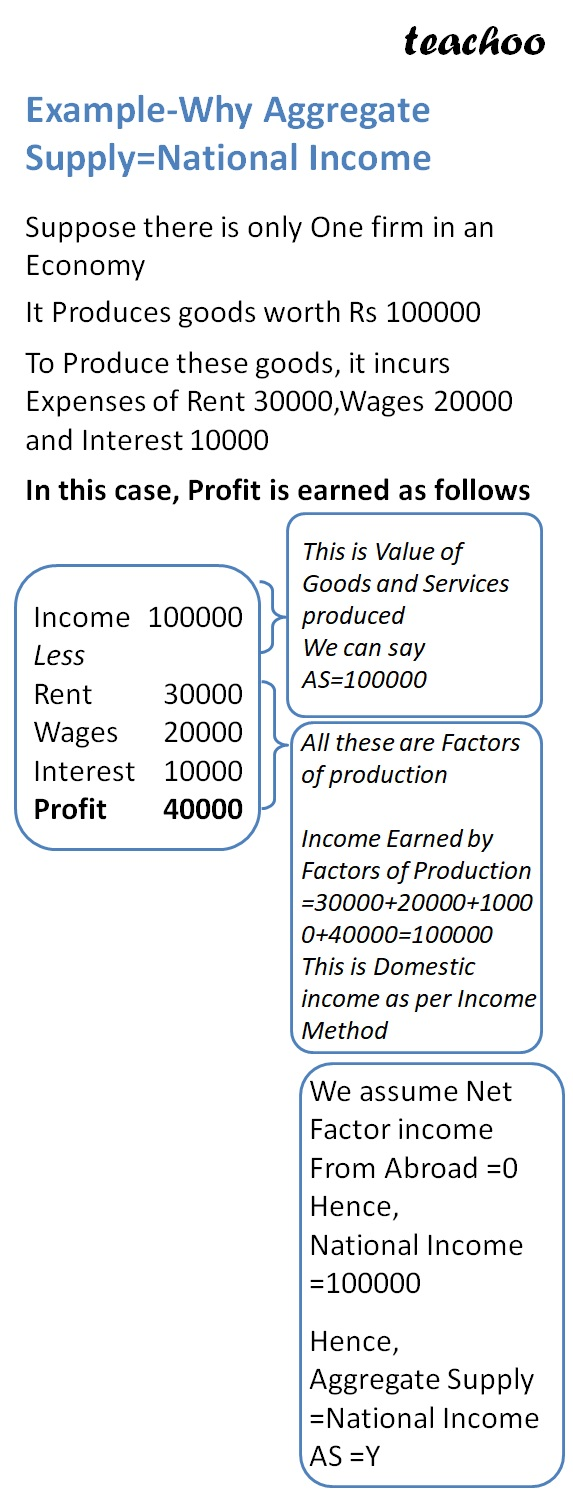Chapter 4 Part 1 - AD,AS and Related Concepts

Economics Class 12
Macroeconomics

## What is Aggregate Supply?

Aggregate Supply means

total money value of goods and services

which all producers in economy

are willing to supply## Why Aggregate Supply is equal to National Income?Second Method

As we know

There are 4 factors of production

Wages, Rent, Interest and Profit

All producers in economy will be supplying either Wages or Rent or Interest or Earning Profit

Aggregate Supply = Wages + Rent + Interest + Profit

Aggregate Supply = Wages + (Rent + Interest + Profit)

Aggregate Supply = Compensation to Employees + operating Surplus

= Net Domestic Product at Factor Cost

= Domestic Income

= National Income

(It is assumed that Net factor Income from Abroad is 0)

### NCERT Questions

No questions in this part

### Other Books

#### Question 1

What is Aggregate Supply?

Learn in your speed, with individual attention - Teachoo Maths 1-on-1 Class

### Transcript

Aggregate Supply Total (All Producers) Value of goods and services which Suppliers as willing to supply Aggregate Supply means Total Value of All goods and Services which all producers of economy are planning to buy at given level period Note Aggregate Supply = National Income AS = Y Why Aggregate Supply is equal to National Income? We know that Firms Sell their Output to Households Also Households provide Factor Services to Firm and Earn Factor income of equal amount Sells Goods & Services This is equal to Aggregate Supply (Value of Output Produced) Provides Factor Services This is equal to Domestic income (Value of Factor Income earned) Since Value of Output Produced=Value of Factor Income Earned Aggregate Supply =Domestic Income Aggregate Supply =National Income (We assume no NFIA) Example-Why Aggregate Supply=National Income Suppose there is only One firm in an Economy It Produces goods worth Rs 100000 To Produce these goods, it incurs Expenses of Rent 30000,Wages 20000 and Interest 10000 In this case, Profit is earned as follows Income Less Rent Wages Interest Profit 100000 30000 20000 10000 40000 This is Value of Goods and Services produced We can say AS=100000 All these are Factors of production Income Earned by Factors of Production =30000+20000+10000+40000=100000 This is Domestic income as per Income Method We assume Net Factor income From Abroad =0 Hence, National Income =100000 Hence, Aggregate Supply =National Income AS =Y